# Write the letters of the word ‘ MATHEMATICS’ which have no line of symmetry.A. SB. MC. ED. YVerified
114.9k+ views
Hint: In this question, we need to find which letter of the word MATHEMATICS has no line of symmetry. First, we need to know about the line of symmetry. That is the line of symmetry is an imaginary line where we can fold the image and have both the halves match exactly. For checking the line of symmetry of an image, we need to draw a horizontal line and then a vertical line through the centre of the image. Using this we can easily find the letter which has no line of symmetry.

Given, MATHEMATICS
Now we need to check which of the given alphabets, that are S, M, E and Y has no line of symmetry.
Let us check the given options one by one whether they have a line of symmetry or not.
First, Let us consider (A), S
Now on drawing a horizontal line,
We get,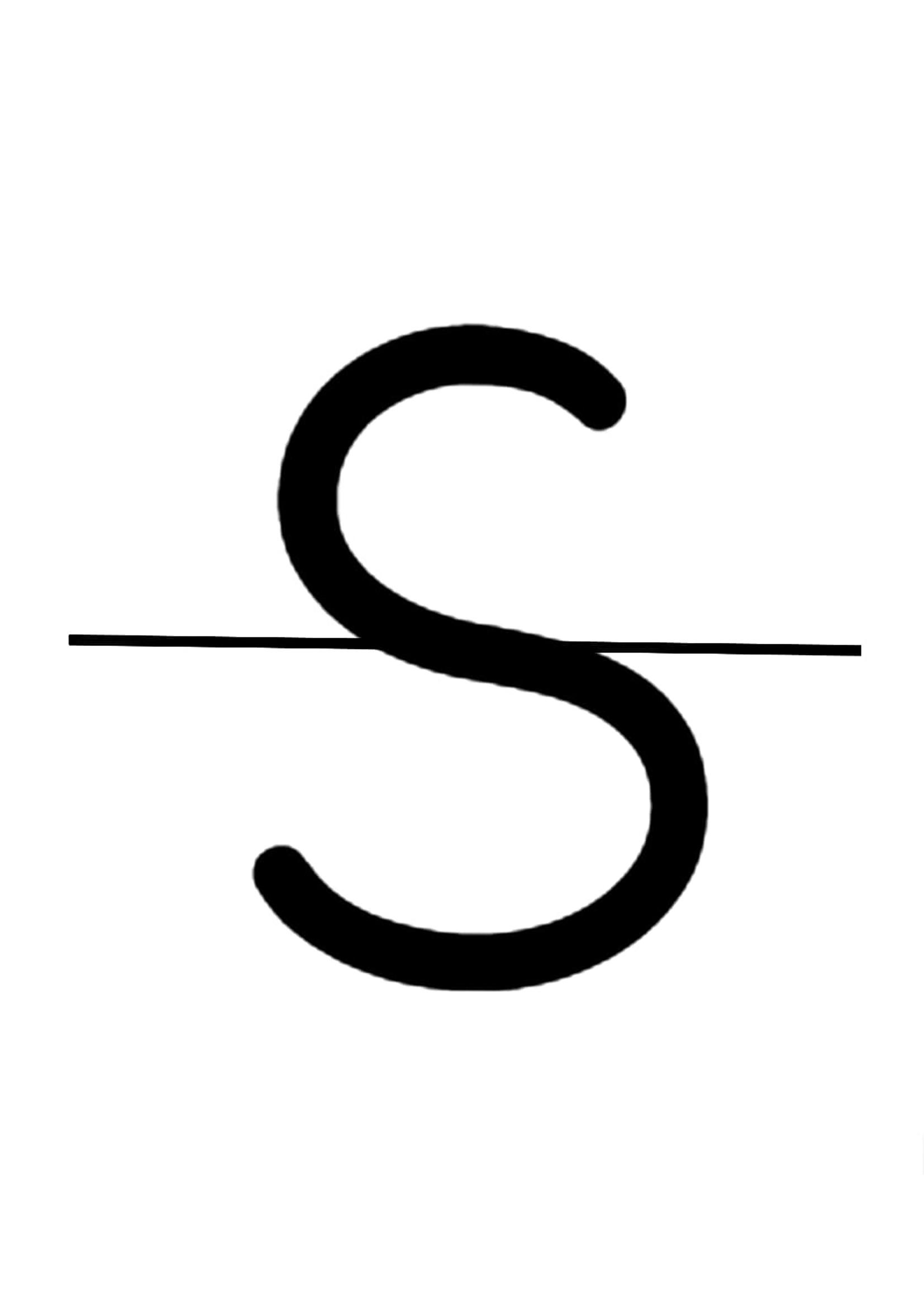We observe that two halves don’t match. So, let us try it by drawing a vertical line .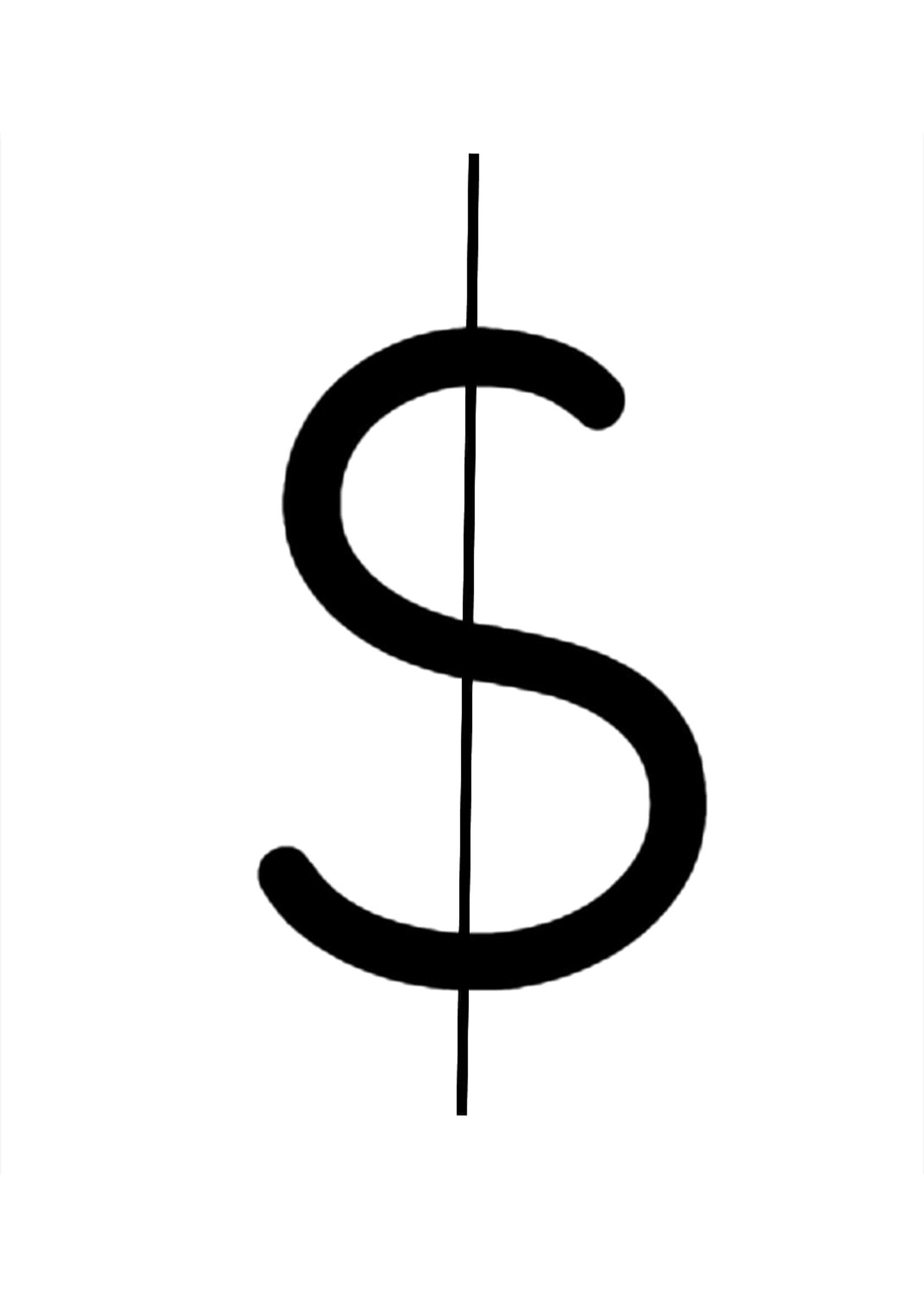Now also two halves don’t match. Hence S has a line of symmetry.
Next let us consider the option (B) M
Now on drawing a horizontal line,
We get,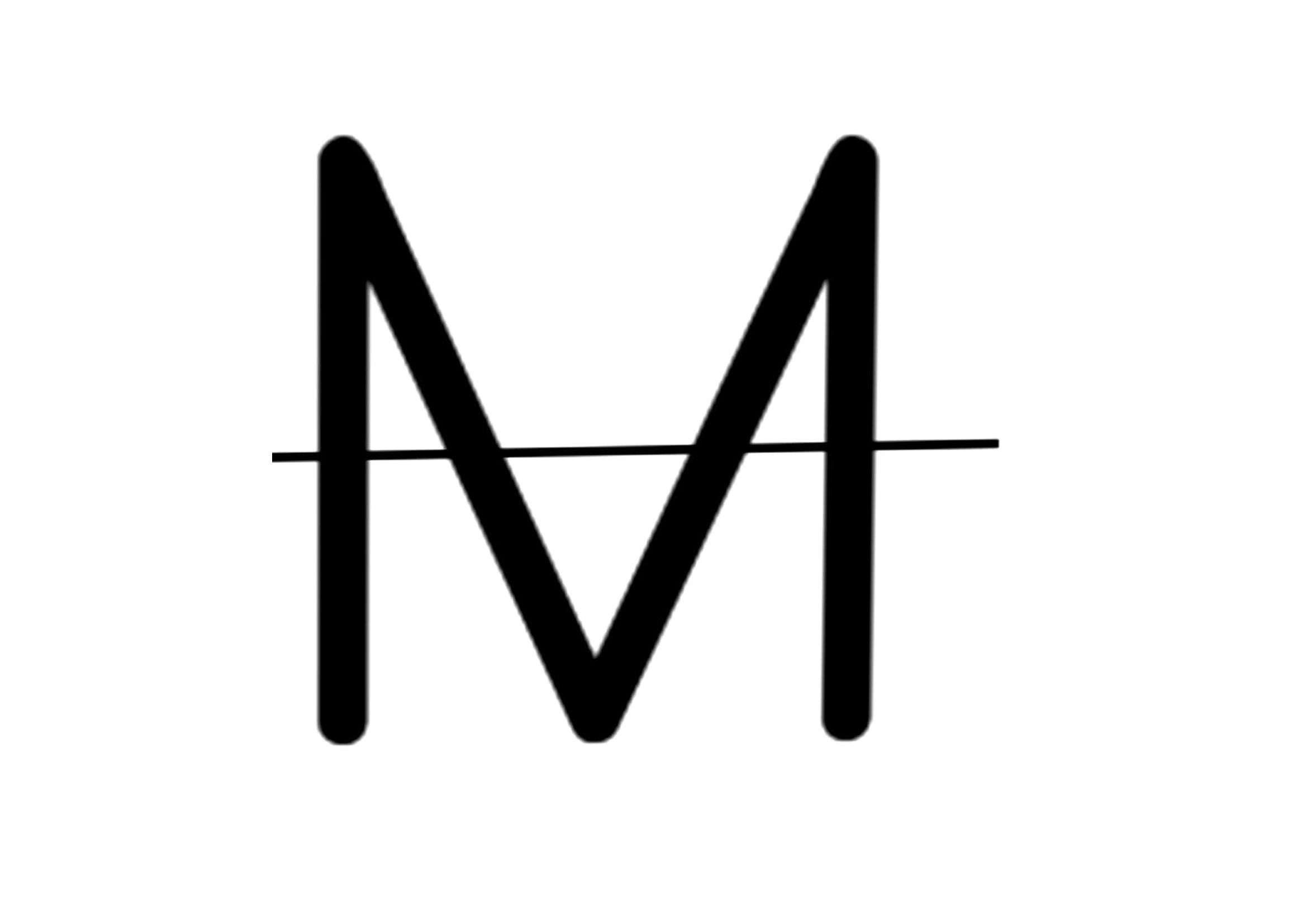We observe that two halves don’t match. So, let us try it by drawing a vertical line .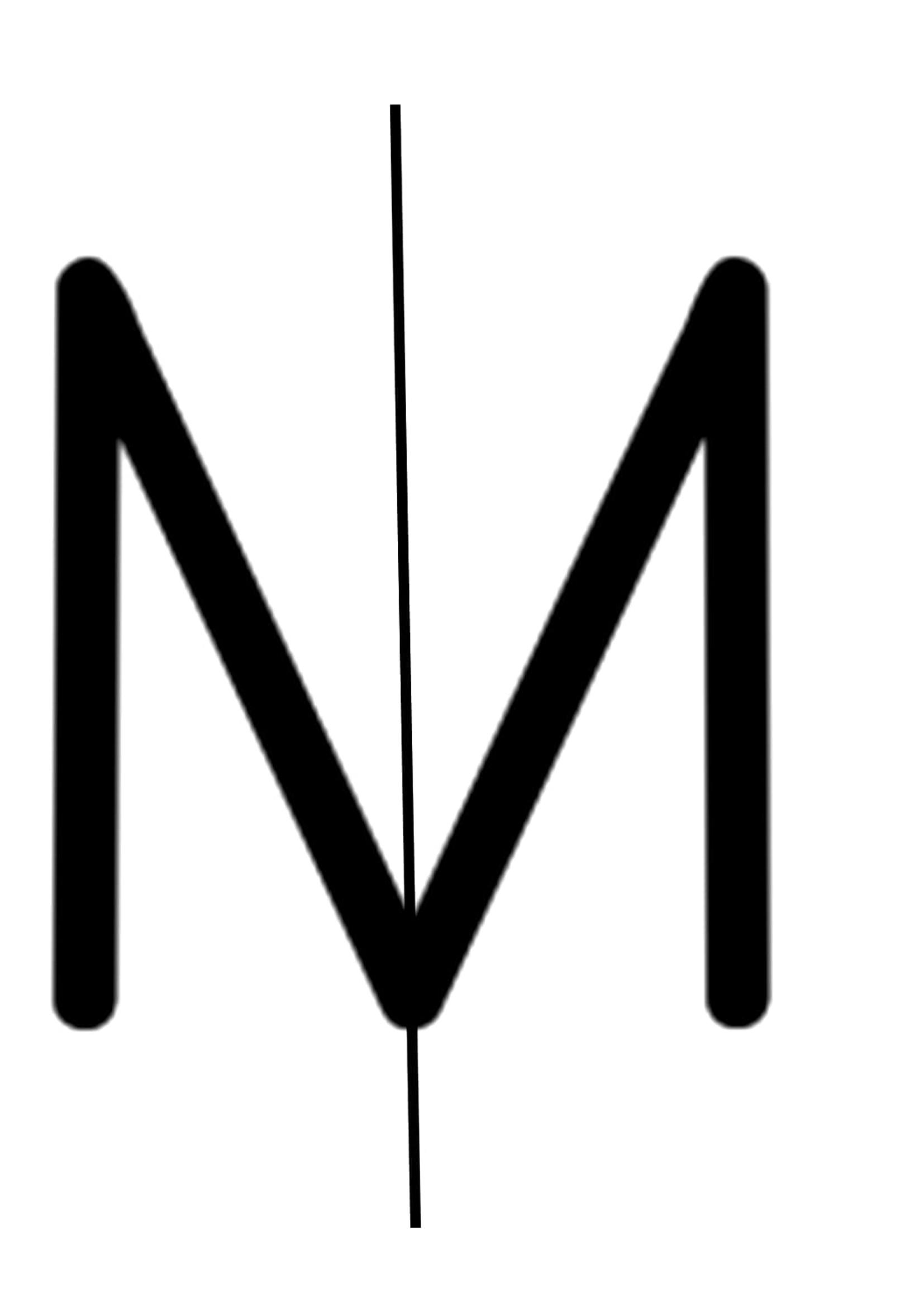We observe that the vertical line divides M into two equal parts. Hence M has a line of symmetry.
Then let us consider the option (C) E
Now on drawing a horizontal line,
We get,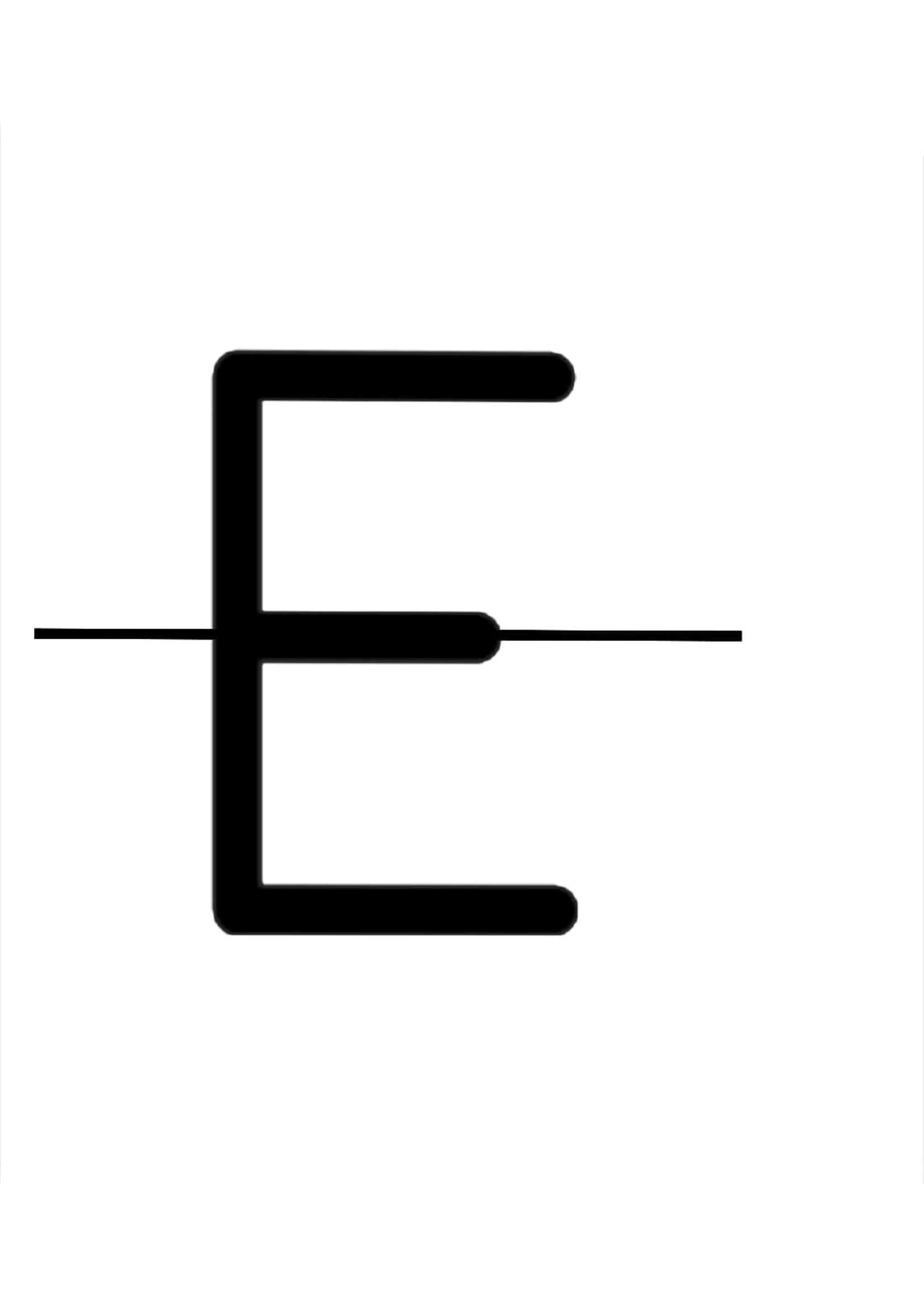We observe that the horizontal line divides E into two equal parts. Hence E has a line of symmetry.
Finally let us check the option (D) Y
Now on drawing a horizontal line,
We get,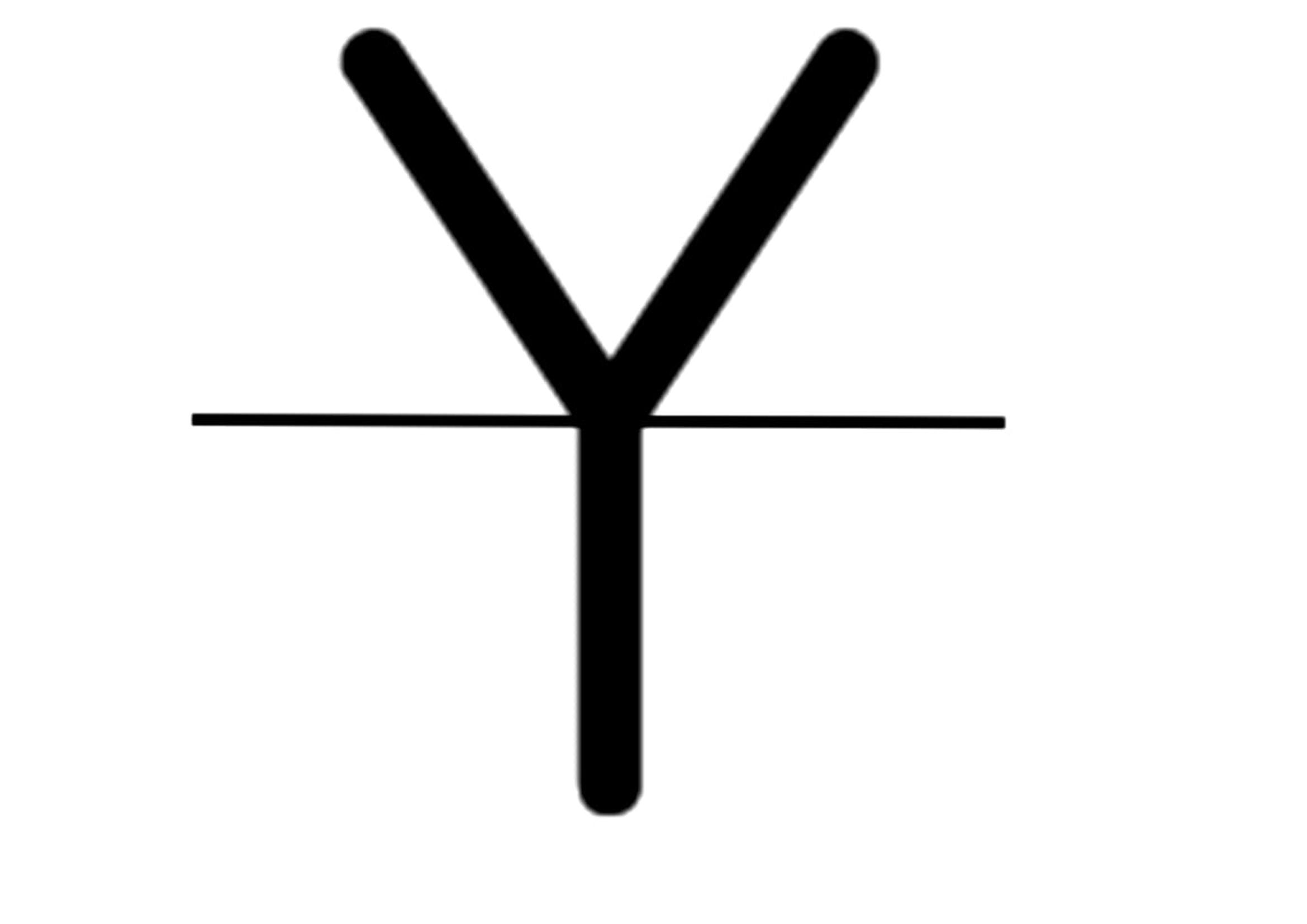We observe that two halves don’t match. So, let us try it by drawing a vertical line .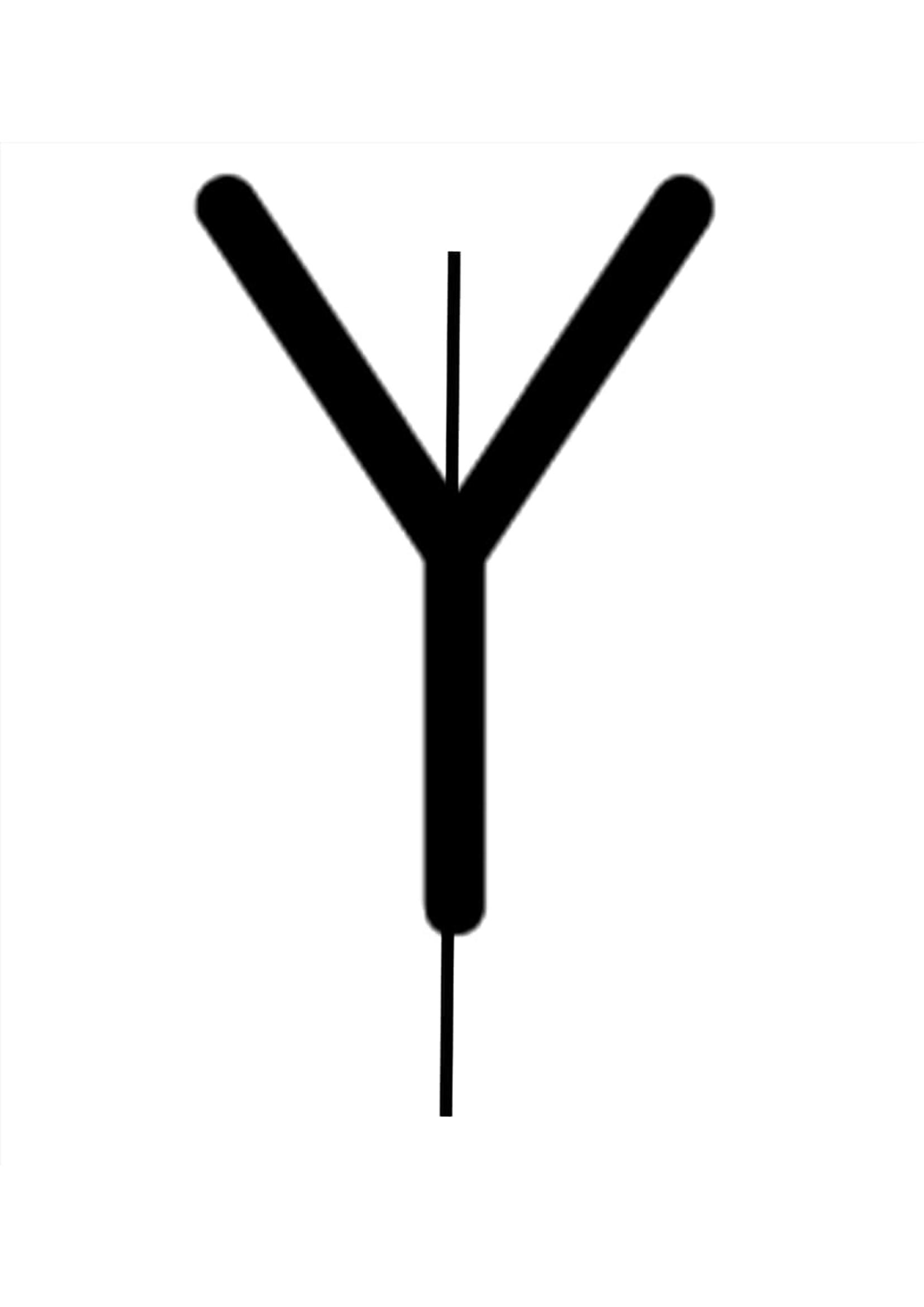We observe that the vertical line divides Y into two equal parts. Hence Y has a line of symmetry.
Thus the letter S has no line of symmetry.